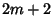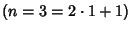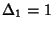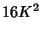## Cyclic Polygon

A cyclic polygon is a Polygon with Vertices upon which a Circle can be Circumscribed. Since every Triangle has a Circumcircle, every Triangle is cyclic. It is conjectured that for a cyclic polygon ofsides,(whereis the Area) satisfies a Monic Polynomial of degree, where(1)(2)

(Robbins 1995). It is also conjectured that a cyclic polygon withsides satisfies one of two Polynomials of degree. The first few values ofare 1, 7, 38, 187, 874, ... (Sloane's A000531).

For Triangles, the Polynomial is Heron's Formula, which may be written(3)

and which is of orderin. For a Cyclic Quadrilateral, the Polynomial is Brahmagupta's Formula, which may be written(4)

which is of orderin. Robbins (1995) gives the corresponding Formulas for the Cyclic Pentagon and Cyclic Hexagon.

References

Robbins, D. P. Areas of Polygons Inscribed in a Circle.'' Discr. Comput. Geom. 12, 223-236, 1994.

Robbins, D. P. Areas of Polygons Inscribed in a Circle.'' Amer. Math. Monthly 102, 523-530, 1995.

Sloane, N. J. A. Sequence A000531 in The On-Line Version of the Encyclopedia of Integer Sequences.'' http://www.research.att.com/~njas/sequences/eisonline.html.